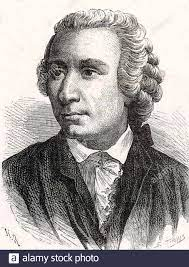Mathematicians have tried in vain to this day to discover some order inthe sequence of prime numbers, and we have reason to believe that it is amystery into which the human mind will never penetrate.

# Understanding these basic definition and statement~

• A sequence is a function whose domain is the set $\mathbb{N}$ of natural numbers.
• A sequence $\left{x_{n}\right}$ is said to converge to a real number $x$, provided that for each $\varepsilon>0$ there exists an integer $N$ such that $n \geq N$ implies that $\left|x_{n}-x\right|<\varepsilon$ In this case we also say that $\left{x_{n}\right}$ converges to $x$, or $x$ is the limit of $\left{x_{n}\right}$, and we write $x_{n} \rightarrow x$, or $\lim {n \Rightarrow \infty} x{n}=x .$ If $\left{x_{n}\right}$ does not converge, it is said to diverge.
• A sequence $\left{x_{n}\right}$ is said to be bounded if the range $\left{x_{n}: n \in \mathbb{N}\right}$ is a bounded set, that is, if there exists $M \geq 0$ such that $\left|x_{n}\right| \leq M$ for all $n \in \mathbb{N}$.
• Bolzano-Weierstrass Theorem: Every bounded sequence has a convergent subsequence.
• Let $\left{x_{n}\right}_{n=1}^{\infty}$ be a sequence and for each $n \in \mathbb{N}$, set
$$y_{n}=\sup \left{x_{k}: k \geq n\right} .$$
The limit superior of $\left{x_{n}\right}$, denoted by $\lim \sup \left{x_{n}\right}$ or $\varlimsup_{n}\left{x_{n}\right}$, is defined by
$$\varlimsup_{n}\left{x_{n}\right}=\inf \left{y_{n}: n \in \mathbb{N}\right}=\inf \left{x: x=\sup \left{x_{k}: k \geq n\right} \text { for some } n \in \mathbb{N}\right}$$

provided that the quantity on the right exists. Likewise we define the limit inferior by
$$\varliminf_{{}\left{x_{n}\right}=\sup \left{x: x=\inf \left{x_{k}: k \geq n\right} \text { for some } n \in \mathbb{N}\right}$$
It is well known that if $\left{x_{n}\right}$ is a sequence, then $\left{x_{n}\right}$ has a limit if and only if the limit superior and the limit inferior exist and are equal.

• A sequence $\left{x_{n}\right}$ of real numbers is said to be a Cauchy sequence if for every $\varepsilon>0$, there is an integer $N$ such that
$$\left|x_{n}-x_{m}\right|<\varepsilon \text { if } n \geq N \text { and } m \geq N$$
Let $\left{x_{n}\right}_{n=1}^{\infty}$ be a sequence and let $\left{n_{k}\right}_{k=1}^{\infty}$ be any sequence of natural numbers such that $n_{1}<n_{2}<n_{3}<\ldots$ The sequence $\left{x_{n_{k}}\right}_{k=1}^{\infty}$ is called a subsequence of $\left{x_{n}\right}_{n=1}^{\infty}$.

# Try the following problem to test yourself！

Problem 1.

Let $\left{x_{n}\right}$ and $\left{y_{n}\right}$ be two real sequences such that
(a) $x_{n} \leq y_{n}$ for all $n$;
(b) $\left{x_{n}\right}$ is increasing;
(c) $\left{y_{n}\right}$ is decreasing.
Show that $\left{x_{n}\right}$ and $\left{y_{n}\right}$ are convergent and
$$\lim {n \rightarrow \infty} x{n} \leq \lim {n \rightarrow \infty} y{n} \text {. }$$
When do we have equality of the limits?

Proof .

Since $\left{y_{n}\right}$ is decreasing, we have $y_{n} \leq y_{1}$ for $n \geq 1 .$ So for any $n \geq 1$ we have $x_{n} \leq y_{n} \leq y_{1}$. This implies that $\left{x_{n}\right}$ is bounded above. Since it is increasing it converges. Similar argument shows that $\left{y_{n}\right}$ is bounded below and therefore converges as well. From (a) we get the desired inequality on the limits. In order to have the equality of the limits we must have $\lim {n \rightarrow \infty} y{n}-x_{n}=0$. This result is useful when dealing with nested intervals in $\mathbb{R}$ and alternating real series.

Problem 2.

Discuss the convergence or divergence of
$$x_{n}=\frac{[\alpha]+[2 \alpha]+\cdots+[n \alpha]}{n^{2}},$$
where $[x]$ denotes the greatest integer less than or equal to the real number $x$, and $\alpha$ is an arbitrary real number.

Proof .

By definition of the greatest integer function $[\cdot]$, we have
$$[x] \leq x<[x]+1$$
for any real number $x$. This will easily imply $x-1<[x] \leq x$. So
$$\frac{(\alpha-1)+(2 \alpha-1)+\cdots+(n \alpha-1)}{n^{2}}<\frac{[\alpha]+[2 \alpha]+\cdots+[n \alpha]}{n^{2}} \leq \frac{\alpha+2 \alpha+\cdots+n \alpha}{n^{2}}$$
or
$$\frac{(1+2+\cdots+n) \alpha-n}{n^{2}}<\frac{[\alpha]+[2 \alpha]+\cdots+[n \alpha]}{n^{2}} \leq \frac{(1+2+\cdots+n) \alpha}{n^{2}} .$$
The algebraic identity $1+2+\cdots+m=\frac{m(m+1)}{2}$ for any natural number $m \geq 1$ gives
$$\frac{\frac{n(n+1)}{2} \alpha-n}{n^{2}}<\frac{[\alpha]+[2 \alpha]+\cdots+[n \alpha]}{n^{2}} \leq \frac{\frac{n(n+1)}{2} \alpha}{n^{2}}$$
or
$$\frac{(n+1) \alpha}{2 n}-\frac{1}{n}<\frac{[\alpha]+[2 \alpha]+\cdots+[n \alpha]}{n^{2}} \leq \frac{(n+1) \alpha}{2 n} .$$
Since
$$\lim {n \rightarrow \infty} \frac{(n+1) \alpha}{2 n}-\frac{1}{n}=\frac{\alpha}{2} \text { and } \lim {n \rightarrow \infty} \frac{(n+1) \alpha}{2 n}=\frac{\alpha}{2},$$
the Squeeze Theorem implies $\lim {n \rightarrow \infty} x{n}=\frac{\alpha}{2}$.

Problem 3.

Show that $\left{x_{n}\right}$ defined by
$$x_{n}=1+\frac{1}{2}+\cdots+\frac{1}{n}$$
is divergent.

Proof .

We have
$$x_{2 n}-x_{n}=\frac{1}{n+1}+\frac{1}{n+2}+\cdots+\frac{1}{2 n}$$
for any $n \geq 1$. So
$$\frac{1}{n+n}+\frac{1}{n+n}+\cdots+\frac{1}{2 n} \leq x_{2 n}-x_{n}$$
or $\frac{1}{2} \leq x_{2 n}-x_{n} .$ This clearly implies that $\left{x_{n}\right}$ fails to be Cauchy. Therefore it diverges.